# Random

tutorial p5.js javascript random

This tutorial introduces the `random()` function and how randomness can be used to create programs that make choices, implement procedural generation, and put together random text.

# Review

Before we jump into the `random()` function, let’s review what we’ve learned so far.

## Functions and Variables

At this point, you should be familiar with functions and variables in P5.js. For example, what does this sketch do?

``````function setup() {
createCanvas(200, 100);
background(32);

var circleSize = 75;
ellipse(width/2, height/2, circleSize)
}
``````

This sketch creates a 200x100 canvas, then draws a dark gray background. It then creates a `circleSize` variable that stores the value `75`, which it uses to draw a circle.

Code Editor

See the Pen by Happy Coding (@KevinWorkman) on CodePen.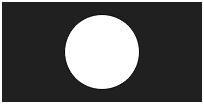## Getting Values from Functions

So far we’ve seen functions that take actions, such as the `createCanvas()` function which creates a canvas, the `background()` function which draws a background, and the `ellipse()` function that draws a circle. But it’s also possible for functions to return a value, which you can then store in a variable and pass in to other functions.

Here’s an example:

``````function setup() {
createCanvas(200, 100);
}

function draw() {
var currentYear = year();
var currentMonth = month();
var currentDay = day();
var currentHour = hour();
var currentMinute = minute();
var currentSecond = second();

var currentDate = currentYear + '-' + nf(currentMonth, 2) + '-' + nf(currentDay, 2);
var currentTime = currentHour + ':' + nf(currentMinute, 2) + ':' + nf(currentSecond, 2);

background(32);

fill(255);
noStroke();
textSize(24);

text(currentDate, 40, 40);
text(currentTime, 40, 70);
}
``````

This sketch uses the time functions like `year()` and `hour()` to get the current date and time. It then uses string concatenation to create text messages that are then displayed on the screen. Also note that this program uses the `draw()` function, which fires 60 times per second.

In other words, this program draws the current date and time to the screen.

Code Editor

See the Pen by Happy Coding (@KevinWorkman) on CodePen.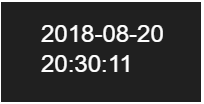# Random

The `random()` function is a little bit like the time functions, only instead of returning a value based on the time, the `random()` function returns a random value every time it’s called.

There are a few different ways to use the `random()` function:

• `random()` returns a value between `0` and `1`.
• `random(max)` returns a value between `0` and `max`.
• `random(min, max)` returns a value between `min` and `max`.

The `random()` function will return a different random value every time you call it. Why is this useful? Let’s see some examples.

## Using Random

Let’s take our circle drawing example and add some randomness to it:

``````function setup() {
createCanvas(200, 200);
background(32);

var circleX = random(width);
var circleY = random(height);
var circleSize = random(10, 100);
ellipse(circleX, circleY, circleSize)
}
``````

This sketch calls `random(width)` to get a random value between `0` and `width`, which it uses for the X position of the circle. Then it calls `random(height)` to get a random value between `0` and `height`, which it uses for the Y position of the circle. Then it calls `random(10, 100)` to get a random value between `10` and `100`, which it uses for the size of the circle. Finally, it uses these values to draw a circle.

Each time the program is run, the calls to `random()` return different randomized values. In other words, this program shows a circle with a random location and size every time it runs!

Code Editor

See the Pen by Happy Coding (@KevinWorkman) on CodePen.

Try running this random circle script and hitting refresh.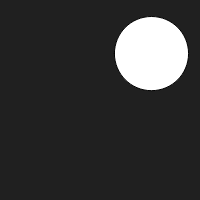Challenge: Notice that the circle can go off the edge of the canvas. Modify this program so the cirlce is always completely inside the bounds of the canvas.

## Random Colors

Similar to how we used the `random()` function to generate a random size and position for our circle, we can also use the `random()` function to come up with random colors.

Remember that colors are generally defined as three values: red, green, and blue, which range in value from `0` to `255` and mix together to form other colors. For example, `fill(64, 128, 255)` will change the fill color to a mix of a little bit of red, some green, and a lot of blue, which ends up being a pale blue color.

To generate a value between `0` and `255`, we can call `random(255)`. We can pass this directly into the `fill()` function to set a random fill color:

``````fill(random(255), random(255), random(255));
``````

Let’s add this line of code to our circle program to give our circle a random color:

``````function setup() {
createCanvas(200, 200);
background(32);

var circleX = random(width);
var circleY = random(height);
var circleSize = random(10, 100);
fill(random(255), random(255), random(255));
ellipse(circleX, circleY, circleSize)
}
``````

Now every time you run the program, it shows a circle with a random location, size, and color.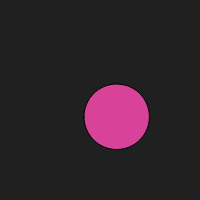Code Editor

See the Pen by Happy Coding (@KevinWorkman) on CodePen.

Try running this random colored random circle program and hitting refresh.

Challenge: Modify this program so it has a random background color.

## Animation

So far, all of our programs draw one thing when they run, which isn’t very interesting. But one of the great things about P5.js is that you can make programs that are interactive and animated.

Remember that P5.js automatically runs any code inside the `draw()` function 60 times per second. We can take advantage of this to create randomized animations.

For example, we can move our circle drawing code so it’s inside the `draw()` function:

``````function setup() {
createCanvas(200, 200);
background(32);
}

function draw(){
var circleX = random(width);
var circleY = random(height);
var circleSize = random(10, 100);
fill(random(255), random(255), random(255));

ellipse(circleX, circleY, circleSize)
}
``````

Now instead of drawing a single circle, our program will draw 60 random circles every second!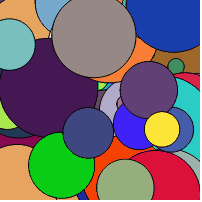Code Editor

See the Pen by Happy Coding (@KevinWorkman) on CodePen.

Challenge: Try randomizing other shapes to create interesting patterns.

## Examples of Random Art

This technique can be used to generate randomized art. Here are a few examples:

# Random Chance

The `random()` function can also be used with `if` statements to make random choices in your code.

Remember that calling `random()` with no arguments returns a random decimal value between `0` and `1`. Every time you call `random()`, you’ll get values like `0.001`, `0.25`, `0.3333`, and `0.99`.

Think about where these values would be on a number line: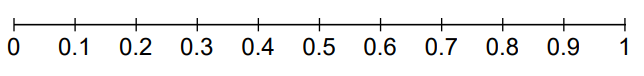Let’s say you wanted to simulate something like a coin toss, where you flip a coin and have a 50% chance for it to land on heads, and a 50% chance to land on tails.

Think about the `0` to `1` number line. If you drew a line at a point on the number line so that 50% of the numbers were on the left of the line and 50% of the numbers were on the right of the line, where would that line be? It would be 50% between `0` and `1`, at `.5`. In other words, of all the numbers you can pick between `0` and `1`, half of them will be less than `.5`, and half of them will be greater than or equal to `.5`.

Going back to the `random()` function: remember that calling `random()` with no arguments returns a decimal number between `0` and `1`. So if we want to flip a coin and have a 50% chance of each option happening, we can compare the value returned from `random()` to `.5`, like this:

``````var randomValue = random();
if(randomValue < 0.5){
}
else{
text("Tails", 25, 55);
}
``````

Read through the code line by line. First we get a random value between `0` and `1`, and we store it in a variable named `randomValue`. Then we compare that value to `.5`. If it’s less than `.5`, we can say the coin flip was heads, otherwise we say the coin flip was tails.

In other words, this code will show “Heads” half the time, and “Tails” the other half of the time.

Here’s a more complete example:

``````function setup() {
createCanvas(200, 100);
background(32);
noStroke();
fill(255);
textSize(18);
text("Click to flip a coin.",25, 55);
}

function mousePressed() {
background(32);
var randomValue = random();
if(randomValue < 0.5){
}
else{
text("Tails", 25, 55);
}
}
``````

This code uses the `mousePressed()` function to execute code every time the user clicks their mouse. In this case, we use the `random()` function along with the `if` statement to show “Heads” or “Tails” whenever the user clicks their mouse.Code Editor

See the Pen by Happy Coding (@KevinWorkman) on CodePen.

Challenge: Create a program that randomly draws heads or tails.

## Multiple Choices

We can use the same kind of logic to choose between more than two possible options.

For example, let’s say we want to simulate rolling dice. A die has 6 sides, so each side has a 1/6 chance to be rolled. 1/6 is 16.666%, so each side has a 16.666% chance of happening.

We want to split our choices up into intervals that each contain 1/6, or 16.666% of the values between `0` and `1`. In other words, we want intervals that are each `0.1666`.

Let’s put together an `if` statement using this logic:

``````var randomValue = random();
if(randomValue < 0.1666){
text("You rolled a 1!", 25, 55);
}
else if(randomValue < 0.3333){
text("You rolled a 2!", 25, 55);
}
else if(randomValue < 0.5){
text("You rolled a 3!", 25, 55);
}
else if(randomValue < 0.6666){
text("You rolled a 4!", 25, 55);
}
else if(randomValue < 0.8333){
text("You rolled a 5!", 25, 55);
}
else{
text("You rolled a 6!", 25, 55);
}
``````

The best way to understand code is to read through it line by line. This code generates a random value between `0` and `1`, and stores it in the `randomValue` variable. Then it checks if `randomValue` is less than `0.1666`, which corresponds to the 16.666% chance of each side. If the value is less than `0.1666` then the code displays `"You rolled a 1!"` on the screen, and the rest of the comparisons are skipped.

However, if the value is greater than `0.1666`, the code then goes to the first `else if` line and checks whether `randomValue` is less than `0.3333`. Keep in mind that if we reach this line, then we know that `randomValue` is not less than `0.1666`. So if it is less than `0.3333`, then we know it’s between `0.1666` and `0.3333`. The space between `0.3333` and `0.1666` is `0.3333 - 0.1666`, which is (approximately) `0.1666`.

The rest of the `if` statement works using a similar logic. If we get passed the `else if(randomValue < 0.3333)` line, then we know the value is greater than or equal to `0.3333`, and we check whether it’s less than `0.5`. And `0.5 - 0.3333` is (approximately) `0.1666`, so there’s an equal chance of rolling a 1, 2, or 3. In fact, there’s an equal chance to roll a 1, 2, 3, 4, 5, or 6!

Try thinking of a few example values between `0` and `1` and walking through the code line by line to understand exactly how the `if` statement works.

Putting it all together, this program simulates rolling dice whenever the user clicks:

``````function setup() {
createCanvas(200, 100);
background(32);
noStroke();
fill(255);
textSize(18);
text("Click to roll the dice.", 20, 55);
}

function mousePressed() {
background(32);
var randomValue = random();
if(randomValue < 0.1666){
text("You rolled a 1!", 25, 55);
}
else if(randomValue < 0.3333){
text("You rolled a 2!", 25, 55);
}
else if(randomValue < 0.5){
text("You rolled a 3!", 25, 55);
}
else if(randomValue < 0.6666){
text("You rolled a 4!", 25, 55);
}
else if(randomValue < 0.8333){
text("You rolled a 5!", 25, 55);
}
else{
text("You rolled a 6!", 25, 55);
}
}
``````
Code Editor

See the Pen by Happy Coding (@KevinWorkman) on CodePen.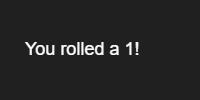Challenge: Create a program that simulates a 20-sided die. Create a program that randomly chooses rock, paper, or scissors whenever the user clicks.

## Random Walkers

We can use the random multiple choice logic to implement one of my favorite algorithms: the random walker.

The random walker is an algorithm designed for exploring a space: stuff like solving a maze, or finding a path from one point to another. The random walker works like this:

• Imagine a grid, like a checkerboard. Each cell of the grid has 4 neighbors: above, below, left, and right neighbors.
• Now imagine that you’re in that grid. To choose where to go next, you randomly choose a neighbor of the random cell.
• Repeat that over and over again to create a path. It’s all random, so sometimes you’ll go backwards, or your path will cross itself. That’s okay!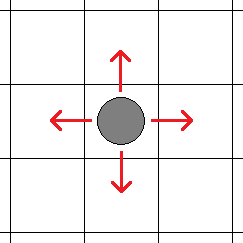This algorithm is not very smart, but if you let a random walker do its thing for a while and draw a path wherever it goes, you’ll get really interesting patterns.

We can create a program that starts in the middle of the screen, and then uses the `random()` function to randomly choose a neighbor every time the `draw()` function is called.

``````var x;
var y;

function setup() {
createCanvas(200, 200);
x = width / 2;
y = width / 2;
background(32);
stroke(255);

for(var i = 0; i < 100000; i++){
draw();
}
}

function draw() {

var randomValue = random();
if(randomValue < .25){
x--;
}
else if(randomValue < .5){
x++;
}
else if(randomValue < .75){
y--;
}
else{
y++;
}

if(x < 0){
x = width;
}
else if(x > width){
x = 0;
}

if(y < 0){
y = height;
}
else if(y > height){
y = 0;
}

point(x, y);
}
``````
Code Editor

See the Pen by Happy Coding (@KevinWorkman) on CodePen.

Also notice that we’re using `if` statements to wrap the line around to the other side if it goes off an edge. If it goes off the top edge, it reappears at the bottom, and vice versa. Same for left and right.

Here are are a few results of letting a random walker take 100,000 steps in a 200x200 grid: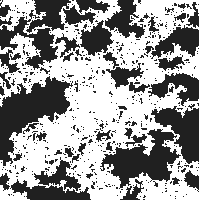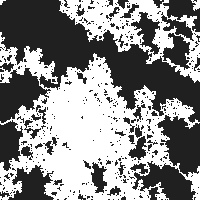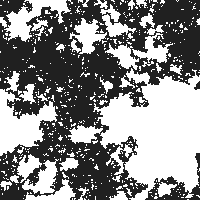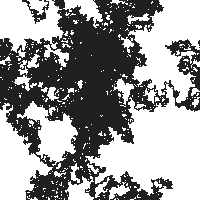This is an example of one of my favorite concepts: emergence is the idea that simple rules can lead to complex behaviors. Think about stuff like ant colonies, or bird flocking, or even how your brain works. They all work using simple rules, but if you observe the whole system, then complicated, unpredictable, and beautiful patterns emerge.

Of course, we can also add colors to make our patterns even more interesting: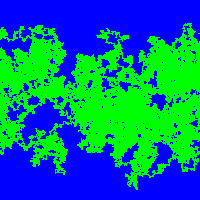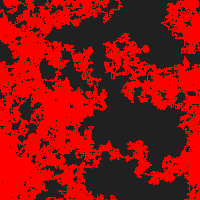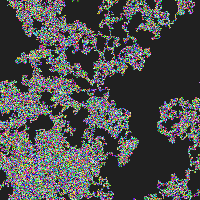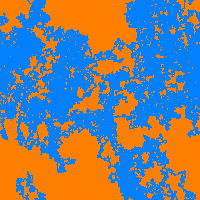We could take it one step further and add some “random walking” logic to the color itself! We start out with variables that hold a red, green, and blue value to be fed into the `stroke()` function. Each step of the simulation, we randomly add or subtract one from each of the variables. This results in a line that changes color as it moves randomly.

``````var x;
var y;

var r;
var g;
var b;

function setup() {
createCanvas(200, 200);

x = width / 2;
y = width / 2;

r = random(0, 255);
g = random(0, 255);
b = random(0, 255);

background(32);
}

function draw() {

// randomly move line
var randomValue = random();
if(randomValue < .25){
x--;
}
else if(randomValue < .5){
x++;
}
else if(randomValue < .75){
y--;
}
else{
y++;
}

// wrap around left and right sides
if(x < 0){
x = width;
}
else if(x > width){
x = 0;
}

// wrap around top and bottom sides
if(y < 0){
y = height;
}
else if(y > height){
y = 0;
}

// randomly change color
r += random(-1, 1);
g += random(-1, 1);
b += random(-1, 1);

// don't let values go outside 0-255 range
r = constrain(r, 0, 255);
g = constrain(g, 0, 255);
b = constrain(b, 0, 255);

stroke(r, g, b);

point(x, y);
}
``````
Code Editor

See the Pen by Happy Coding (@KevinWorkman) on CodePen.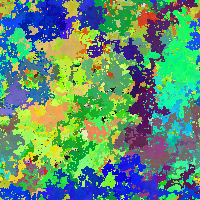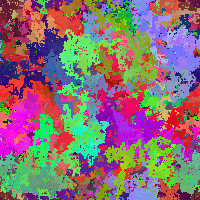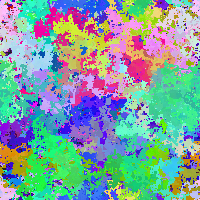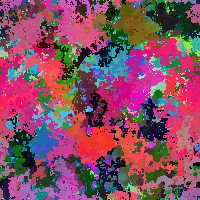Try playing with the colors a bit to create more interesting patterns: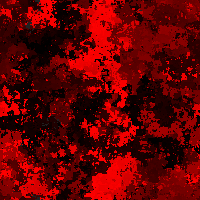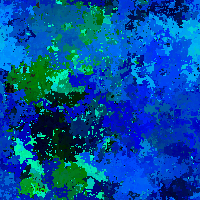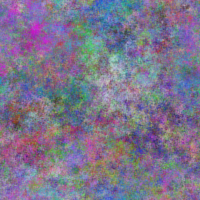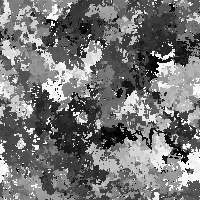Challenge: Try modifying the thickness of the line, or change how much the line moves each step.

# Procedural Generation

The `random()` function is at the core of a technique called procedural generation. Procedural generation uses randomness to automatically generate content, which is useful in video games, movies, and art.

For example, let’s say we want to procedurally generate a flower. We can start with a basic design for a flower, like this: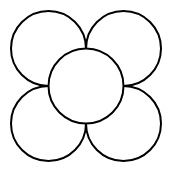We can create this flower by drawing 4 circles for petals, and then a fifth circle for a center.

``````function setup() {
createCanvas(200, 200);

strokeWeight(2);

var petalSize = 75;
var flowerCenterX = width/2;
var flowerCenterY = width/2;

ellipse(flowerCenterX - petalSize/2, flowerCenterY - petalSize/2, petalSize, petalSize);
ellipse(flowerCenterX + petalSize/2, flowerCenterY - petalSize/2, petalSize, petalSize);
ellipse(flowerCenterX - petalSize/2, flowerCenterY + petalSize/2, petalSize, petalSize);
ellipse(flowerCenterX + petalSize/2, flowerCenterY + petalSize/2, petalSize, petalSize);

ellipse(flowerCenterX, flowerCenterY, petalSize, petalSize);
}
``````
Code Editor

See the Pen by Happy Coding (@KevinWorkman) on CodePen.

Notice that we’re using variables to store the size and position. This will come in handy in a second.

Now that we have this code, we can make it more interesting by adding some randomness to it. Let’s use the `random()` function to generate a random size and position:

``````function setup() {
createCanvas(200, 200);

strokeWeight(2);

var petalSize = random(10, 50);
var flowerCenterX = random(width);
var flowerCenterY = random(height);
var r = random(255);
var g = random(255);
var b = random(255);

fill(r, g, b);
ellipse(flowerCenterX - petalSize/2, flowerCenterY - petalSize/2, petalSize, petalSize);
ellipse(flowerCenterX + petalSize/2, flowerCenterY - petalSize/2, petalSize, petalSize);
ellipse(flowerCenterX - petalSize/2, flowerCenterY + petalSize/2, petalSize, petalSize);
ellipse(flowerCenterX + petalSize/2, flowerCenterY + petalSize/2, petalSize, petalSize);

fill(r/2, g/2, b/2);
ellipse(flowerCenterX, flowerCenterY, petalSize, petalSize);
}
``````
Code Editor

See the Pen by Happy Coding (@KevinWorkman) on CodePen.

Now our code procedurally generates a different random flower every time the program runs.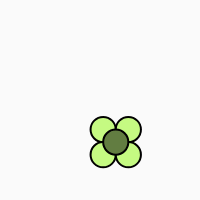Of course, we can put all of this in our `draw()` function to show a bunch of random flowers:

``````function setup() {
createCanvas(200, 200);
frameRate(5);
}

function draw(){
var petalSize = random(10, 50);
var flowerCenterX = random(width);
var flowerCenterY = random(height);
var r = random(255);
var g = random(255);
var b = random(255);

fill(r, g, b);
ellipse(flowerCenterX - petalSize/2, flowerCenterY - petalSize/2, petalSize, petalSize);
ellipse(flowerCenterX + petalSize/2, flowerCenterY - petalSize/2, petalSize, petalSize);
ellipse(flowerCenterX - petalSize/2, flowerCenterY + petalSize/2, petalSize, petalSize);
ellipse(flowerCenterX + petalSize/2, flowerCenterY + petalSize/2, petalSize, petalSize);

fill(r/2, g/2, b/2);
ellipse(flowerCenterX, flowerCenterY, petalSize, petalSize);
}
``````
Code Editor

See the Pen by Happy Coding (@KevinWorkman) on CodePen.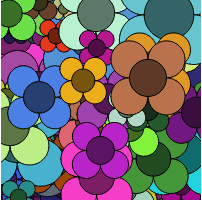Challenge: Modify the flower drawing code to draw more realistic flowers. Or draw something else, like faces or animals.

We could also take this one step further and randomly generate a garden:

``````function setup() {
createCanvas(500, 500);

var skyB = random(128, 255);
var skyG = random(skyB);
var skyR = random(skyG);
background(skyR, skyG, skyB);

var groundG = random(128, 255);
var groundB = random(groundG);
var groundR = random(groundB);

noStroke();
fill(groundR, groundG, groundB);
rect(0, height/2, width, height/2);

for(var i = 0; i < 25; i++){
drawRandomFlower();
}
}

function drawRandomFlower(){
var petalSize = random(10, 20);
var flowerCenterX = random(width);
var flowerCenterY = random(height/2 + petalSize, height);
var r = random(255);
var g = random(255);
var b = random(255);

fill(r, g, b);
ellipse(flowerCenterX - petalSize/2, flowerCenterY - petalSize/2, petalSize, petalSize);
ellipse(flowerCenterX + petalSize/2, flowerCenterY - petalSize/2, petalSize, petalSize);
ellipse(flowerCenterX - petalSize/2, flowerCenterY + petalSize/2, petalSize, petalSize);
ellipse(flowerCenterX + petalSize/2, flowerCenterY + petalSize/2, petalSize, petalSize);

fill(r/2, g/2, b/2);
ellipse(flowerCenterX, flowerCenterY, petalSize, petalSize);
}
``````
Code Editor

See the Pen by Happy Coding (@KevinWorkman) on CodePen.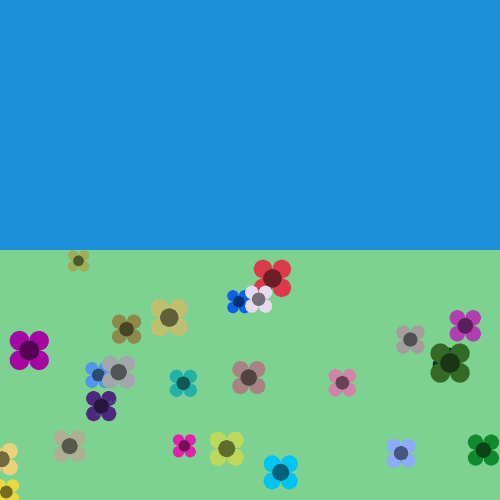Now our code uses a `for` loop to draw 25 random flowers. It also draws a randomly colored sky and ground, and the flower drawing code has been modified so all of the flowers show up on the ground instead of in the sky.

Challenge: Add stars or clouds to the sky. Add trees or animals to the garden.

## Examples of Procedural Generation

Many video games use procedural generation. Here are a few that I can think of:

Many films also use procedural generation, specifically for things like background scenery and large crowd scenes. Here is a partial list of movies that have used procedural generation.

Here are a few Twitter bots that use procedural generation:

# Text Generation

Another way to use the `random()` function is to feed it an array. When you pass in an array, the `random()` function will return a random element from inside that array. Here’s a simple example:

``````var myArray = ['lions', 'tigers', 'bears'];
var myElement = random(myArray);
``````

This code creates an array and stores it in the `myArray` variable. Then it passes that array into the `random()` function, which chooses a random element from the array and returns it. That return value is then stored in the `myElement` variable.

We can use this to randomly generate text:

``````function setup() {
createCanvas(200, 100);
textSize(24);
background(224);

var myArray = ['lions', 'tigers', 'bears'];
var myElement = random(myArray);
text(myElement, 25, 55);
}
``````
Code Editor

See the Pen by Happy Coding (@KevinWorkman) on CodePen.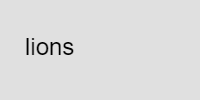We can expand this and use string concatenation (that’s a fancy way of saying the `+` operator) to randomly generate more complicated messages.

``````var helloArray = ['Hello', 'Hey', 'Hi'];
var worldArray = ['world', 'everyone', 'all']

function setup() {
createCanvas(200, 100);
textSize(24);
frameRate(1);
}

function draw(){
background(224);

var randomHello = random(helloArray);
var randomWorld = random(worldArray);
var helloWorldMessage = randomHello + ' ' + randomWorld + '!'
text(helloWorldMessage, 25, 55);
}
``````
Code Editor

See the Pen by Happy Coding (@KevinWorkman) on CodePen.

In this code, we’re creating arrays that hold words that can be used in place of `"hello"` and `"world"`. Then we’re calling the `random()` function to choose random words, which we combine together using the `+` concatenation operator. The result is a random “hello world” message.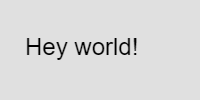We can string more words together to form longer sentences. This approach is similar to mad libs, where you choose random words that go into a larger message or story.

``````var adjectives = ['quick', 'slow', 'lazy', 'hungry'];
var colors = ['brown', 'green', 'poka-dotted'];
var animals = ['fox', 'dog', 'cat', 'lizard'];
var actions = ['jumps over', 'runs around', 'high-fives'];

function setup() {
createCanvas(200, 200);
textSize(24);
frameRate(.25);
}

function draw(){
background(224);

var colorOne = random(colors);
var animalOne = random(animals);
var action = random(actions);
var animalTwo = random(animals);
var sentence = 'The ' + adjectiveOne + ' ' + colorOne + ' ' + animalOne +
' ' + action + ' the ' + adjectiveTwo + ' ' + animalTwo + '!';

text(sentence, 10, 0, width-20, height);
}
``````
Code Editor

See the Pen by Happy Coding (@KevinWorkman) on CodePen.

This code breaks down the sentence “the quick brown fox jumps over the lazy dog” into adjectives, colors, animals, and actions. It then uses the `random()` function to come up with a random version of the sentence.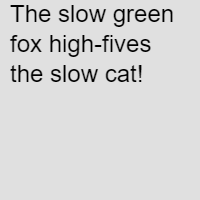Challenge: Creare your own mad lib story. Write a program that generates random song lyrics based on songs from your favorite musician.

# Other Random Functions

This tutorial introduced the `random()` function, but there are other functions related to randomness in P5.js.

• `randomSeed()` can be used to make randomness more predictable. Calling the `randomSeed()` function causes the `random()` function to return the same sequence of random values. Try adding `randomSeed(1)` to the beginning of one of our programs to see what I mean. This can be useful for debugging.

• `randomGaussian()` returns a random value within a bell curve. This can be useful for generating more realistic random values.

• `noise()` returns a random value based on Perlin noise. This can be useful for generating natural patterns and textures.

# What’s Next?

This tutorial was meant to introduce some basic examples of using the `random()` function, but there are a ton of other things you can do with it.

Try building on top of the examples above. Come up with interesting patterns, or write a program that procedurally generates drawings of your favorite animal. Take our garden program above and draw your own randomized scene.

Share what you come up with on the forum!